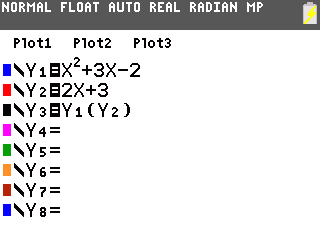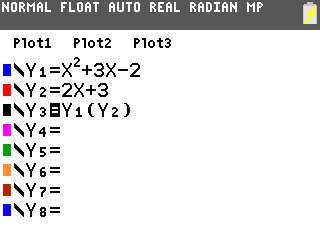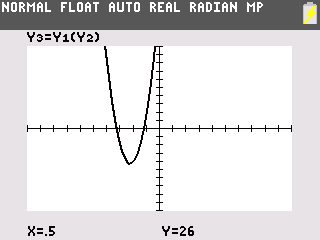# Knowledge Base

## Solution 34417: Evaluating Composite Functions for a Specific Value of X on the TI-83 Plus and TI-84 Plus Family.

### How can I evaluate the composition of two functions given a specific x-value on the TI-83 Plus and TI-84 Plus Family?

In order to evaluate the composition of two functions, given a specific value of x, refer to the following example.

Example - Find the composition of the following two functions when x = .5:
f(x) = x2 + 3x - 2
g(x) = 2x + 3

Please Note: Many textbooks indicate function compositions using the notation f(g(x)) or (f ° g)(x).

Solution: Let Y1 = f(x) and Y2 = g(x)

• Press [Y=] to go to the Y= Editor screen.
• Input x2 + 3x - 2 into the Y1 slot, then press [ENTER].
• Input 2x + 3 into the Y2 slot, then press [ENTER].
• Press [VARS], arrow right to Y-VARS, and select 1:Function from the Y-VARS menu.
• Press 1: Y1 then press [( ].
• Press [VARS], arrow right to Y-VARS, and select 1:Function from the Y-VARS menu.
• Press 2:Y2 then press [ )] [ENTER].

The Y3 slot should read Y3 = Y1(Y2).• Arrow over to the = sign and arrow up 3 times to select the '=' to the right of Y1, then press [ENTER] to disable the function from being graphed.

Please Note: The black square surrounding the '=' disappears once the function is disabled.

• Arrow down once and press [ENTER] to disable the Y2 function from being graphed as well.• Press [GRAPH] to graph the composite function.
• Press [TRACE] then input .5 and press [ENTER].The coordinates are displayed, even if CoordOff format is selected. The y-value of 26 is the solution to the problem.

Please see the TI-83 Plus and TI-84 Plus Family guidebooks for additional information.